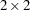The FREQ Procedure

## Example 3.5 Analysis of a 2x2 Contingency Table

This example computes chi-square tests and Fisher’s exact test to compare the probability of coronary heart disease for two types of diet. It also estimates the relative risks and computes exact confidence limits for the odds ratio.

The data set FatComp contains hypothetical data for a case-control study of high fat diet and the risk of coronary heart disease. The data are recorded as cell counts, where the variable Count contains the frequencies for each exposure and response combination. The data set is sorted in descending order by the variables Exposure and Response, so that the first cell of thetable contains the frequency of positive exposure and positive response. The FORMAT procedure creates formats to identify the type of exposure and response with character values.

```proc format;
value ExpFmt 1='High Cholesterol Diet'
0='Low Cholesterol Diet';
value RspFmt 1='Yes'
0='No';
run;
```
```data FatComp;
input Exposure Response Count;
label Response='Heart Disease';
datalines;
0 0  6
0 1  2
1 0  4
1 1 11
;
```
```proc sort data=FatComp;
by descending Exposure descending Response;
run;
```

In the following PROC FREQ statements, ORDER=DATA option orders the contingency table values by their order in the input data set. The TABLES statement requests a two-way table of Exposure by Response. The CHISQ option produces several chi-square tests, while the RELRISK option produces relative risk measures. The EXACT statement requests the exact Pearson chi-square test and exact confidence limits for the odds ratio.

```proc freq data=FatComp order=data;
format Exposure ExpFmt. Response RspFmt.;
tables Exposure*Response / chisq relrisk;
exact pchi or;
weight Count;
title 'Case-Control Study of High Fat/Cholesterol Diet';
run;
```

The contingency table in Output 3.5.1 displays the variable values so that the first table cell contains the frequency for the first cell in the data set (the frequency of positive exposure and positive response).

Output 3.5.1 Contingency Table
 Case-Control Study of High Fat/Cholesterol Diet

The FREQ Procedure

Frequency
Percent
Row Pct
Col Pct
Table of Exposure by Response
Exposure Response(Heart Disease)
Yes No Total
High Cholesterol Diet
 11 47.83 73.33 84.62
 4 17.39 26.67 40
 15 65.22
Low Cholesterol Diet
 2 8.7 25 15.38
 6 26.09 75 60
 8 34.78
Total
 13 56.52
 10 43.48
 23 100

Output 3.5.2 displays the chi-square statistics. Because the expected counts in some of the table cells are small, PROC FREQ gives a warning that the asymptotic chi-square tests might not be appropriate. In this case, the exact tests are appropriate. The alternative hypothesis for this analysis states that coronary heart disease is more likely to be associated with a high fat diet, so a one-sided test is desired. Fisher’s exact right-sided test analyzes whether the probability of heart disease in the high fat group exceeds the probability of heart disease in the low fat group; because this-value is small, the alternative hypothesis is supported.

The odds ratio, displayed in Output 3.5.3, provides an estimate of the relative risk when an event is rare. This estimate indicates that the odds of heart disease is 8.25 times higher in the high fat diet group; however, the wide confidence limits indicate that this estimate has low precision.

Output 3.5.2 Chi-Square Statistics
Statistic DF Value Prob
Chi-Square 1 4.9597 0.0259
Likelihood Ratio Chi-Square 1 5.0975 0.0240
Continuity Adj. Chi-Square 1 3.1879 0.0742
Mantel-Haenszel Chi-Square 1 4.7441 0.0294
Phi Coefficient   0.4644
Contingency Coefficient   0.4212
Cramer's V   0.4644

Pearson Chi-Square Test
Chi-Square 4.9597
DF 1
Asymptotic Pr > ChiSq 0.0259
Exact Pr >= ChiSq 0.0393

Fisher's Exact Test
Cell (1,1) Frequency (F) 11
Left-sided Pr <= F 0.9967
Right-sided Pr >= F 0.0367

Table Probability (P) 0.0334
Two-sided Pr <= P 0.0393

Output 3.5.3 Relative Risk
Estimates of the Relative Risk (Row1/Row2)
Type of Study Value 95% Confidence Limits
Case-Control (Odds Ratio) 8.2500 1.1535 59.0029
Cohort (Col1 Risk) 2.9333 0.8502 10.1204
Cohort (Col2 Risk) 0.3556 0.1403 0.9009

Odds Ratio (Case-Control Study)
Odds Ratio 8.2500

Asymptotic Conf Limits
95% Lower Conf Limit 1.1535
95% Upper Conf Limit 59.0029

Exact Conf Limits
95% Lower Conf Limit 0.8677
95% Upper Conf Limit 105.5488Previous Page | Next Page | Top of Page